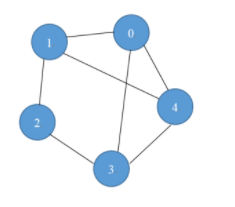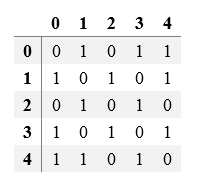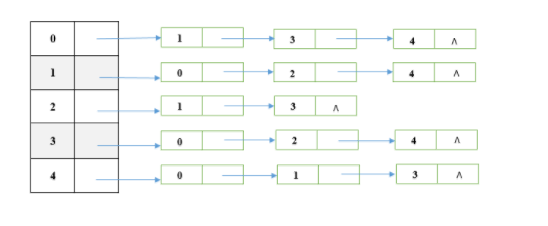# 一、链表

## 循环队列的实现

#include<iostream>
#include <string>
using namespace std;

template <typename T>
class Myloopqueue {
private:
T *queue;//存储用的数组
int capacity;//存放个数
int tail;//指向最后进来的元素
public:
Myloopqueue(int a);//无参构造
Myloopqueue();//有参构造
~Myloopqueue();//析构
bool isEmpty();//判断空
int getSize();//返回个数
bool push(T a);//入队
bool pop();//出队
T top();//显示队首
};

template<typename T>
Myloopqueue<T>::Myloopqueue(int a) :head(0), tail(0), capacity(a), queue(nullptr) {
queue = new T[capacity];
}

template<typename T>
Myloopqueue<T>::~Myloopqueue() {
delete[] queue;
}

template<typename T>
bool Myloopqueue<T>::isEmpty() {
return true;
else
return false;
}

template<typename T>
int Myloopqueue<T>::getSize() {
return (tail - head + capacity) % capacity;
}

template<typename T>
bool Myloopqueue<T>::push(T a) {
if ((tail  + 1) % capacity == head)
return false;
tail = (tail + 1) % capacity;
queue[tail] = a;
return true;
}

template<typename T>
bool Myloopqueue<T>::pop() {
return false;
return true;
}

template<typename T>
T Myloopqueue<T>::top() {
}

int main()
{
Myloopqueue<string> queue(6);
queue.push("one");
queue.push("two");
queue.push("three");
queue.push("four");
queue.push("five");
cout << "队列长度" << queue.getSize() << endl;
while (!queue.isEmpty())
{
cout << queue.top() << endl;
queue.pop();
}
system("pause");
return 0;

}



# 三、图

## 图的两种存储结构

### 邻接矩阵### 邻接表## 1.图的遍历算法操作

### 深度优先搜索遍历DFS

void DFTCore(const vector<vector<int> >& Graph, int k, vector<bool> &visited)
{
if (visited[k] == 1)
return;
cout << k << endl;
visited[k] = 1;
for (int i = 0; i < Graph.size(); ++i)
{
if (Graph[k][i] != -1)
DFTCore(Graph, i, visited);
}
return;
}
void DFT(const vector<vector<int> >& Graph)
{
int num_vertex = Graph.size();
vector<bool> visited(num_vertex, false);
for (int i = 0; i < num_vertex; ++i)
DFTCore(Graph, i, visited);
return;
}
void addEdge(const int& startP, const int& endP, const int& weight, vector<vector<int> >& Graph)
{
Graph[startP][endP] = weight;
}

int main()
{
vector<vector<int> > Graph(6, vector<int>(6, -1));
DFT(Graph);
system("pause");
return 0;
}

### 广度优先搜索遍历BFS

• 任务图中一个顶点访问，入队，并将这个顶点标记为已访问。
• 当队列不空时循环执行：出队，依次检查出队顶点的所有邻接顶点，访问没有被访问过的邻接顶点并将其入队。
• 当队列为空时跳出循环，广度优先搜索即完成。
void BFTCore(const vector<vector<int> >& Graph, int k, vector<bool> &visited)
{
if (visited[k] == true)
return;
queue<int> q;
q.push(k);
while (!q.empty())
{
int value = q.front();
q.pop();
if (visited[value] == false)
{
visited[value] = true;
cout << value << endl;
for (int i = 0; i < Graph.size(); ++i)
{
if (Graph[value][i] != -1 && visited[i] == false)
q.push(i);
}
}
}
return;
}
void BFT(const vector<vector<int> >& Graph)
{
int num_vertex = Graph.size();
vector<bool> visited(num_vertex, false);
//之所以循环，是防止那种图不相连的情况
for (int i = 0; i < num_vertex; ++i)
BFTCore(Graph, i, visited);
return;
}
void addEdge(const int& startP, const int& endP, const int& weight, vector<vector<int> >& Graph)
{
Graph[startP][endP] = weight;
}

int main()
{
vector<vector<int> > Graph(6, vector<int>(6, -1));
BFT(Graph);
system("pause");
return 0;
}

## 2.最短路径算法

### 迪杰斯特拉算法(O(N^2))

Djkstra算法是求解单源（起始点固定）最短路径问题的一种经典方法，它采用了贪心策略（其实我觉得也是动态规划），可以求得图中的一个点到其他所有点的距离，计算复杂度是 O(E|V|)，如果采用最小堆优化可以达到O(ElogV )。算法的整体思想是将顶点分成两类：已经构成最短路的点的集合V1和没有构成最短路的点的集合V2。我们将dist[i]设置为第 i个点到V1的距离，每次将V2中取距离V1集合最短的点P放入V1中，同时因为P被放入了V1，那么其他点到V1的最短路就有可能通过P了，所以我们更新所有集合V2内的点j到V1的距离dist[j] = min(  dist[j], dist[i_P] + G[i_P][j]  )，其中i_P表示P的下标， G[i_P][j]  表示图中P到j的距离。

https://blog.csdn.net/qq_30911665/article/details/78130709

#include <iostream>
#include<vector>
#include<queue>
using namespace std;
#define MAX_PATH 999999
int shortestId(const vector<int>& dist, const vector<bool>& isShortest) //寻找当前未放入最短路径集合的所有ID中路径最短的ID号
{
int min_dist = INT_MAX;
int min_ID = -1;
for (int i = 0; i < dist.size(); i++)
{
if (false == isShortest[i]) {
if (dist[i] < min_dist) {
min_dist = dist[i];
min_ID = i;
}
}
}
return min_ID;
}
vector<int> Djkstra(const vector<vector<int> >& Graph)
{
int num_vertex = Graph.size();
vector<bool> isShortest(num_vertex, false); //初始化只有第一个顶点（index = 0）被放入最短路的ID集合中
isShortest = true;
vector<int> dist(num_vertex, INT_MAX); //dist[i]表示当前节点 i+1(下标i)到最短路的id集合中所有点的最短距离
dist = 0;

for (int i = 1; i < num_vertex; i++)
{
if (Graph[i] <INT_MAX) //初始化dist，所有不与1号节点（下标0）相连的设置为正无穷
dist[i] = Graph[i];
}
for (int i = 0; i < num_vertex - 1; i++) {
int id = shortestId(dist, isShortest); //在所有非最短路的点集合中找到距离最短路集合最近的点，放入最短路集合
isShortest[id] = true;
for (int j = 0; j < num_vertex; j++) { //将 id放入最短路集合后，更新所有集合外的元素的距离，他们有可能有通过id的更短路
if (!isShortest[j]) {
//这个地方导致下面的Graph初始化的时候不能设置为INT_MAX，因为有可能相加之后超出INT_MAX
dist[j] = min(dist[j], dist[id] + Graph[id][j]);
}
}
}
return dist;
}
void addEdge(const int& startP, const int& endP, const int& weight, vector<vector<int> >& Graph)
{
Graph[startP][endP] = weight;
//Graph[endP][startP] = weight;
}

int main()
{
vector<vector<int> > Graph(6, vector<int>(6, MAX_PATH));
/*   for(int i =0 ; i < Graph.size(); i++)
{
for(int j = 0; j < Graph.size(); j++)
cout << Graph[i][j] << "\t";
cout <<endl;
}*/
vector<int> shortestDist = Djkstra(Graph);
for (int i = 0; i <shortestDist.size(); i++)
cout << shortestDist[i] << endl;
system("pause");
return 0;
}


## 3.最小生成树

### 普里姆算法(O(N^2))


#include <iostream>
#include<vector>
using namespace std;
pair<int, int> GetShortestEdge(const vector<vector<int> >& Graph, const vector<bool>& isIncluded)//求当前在MST之外距离MST最近的点的id
{
int minDist = INT_MAX;
pair<int, int> minEdge;
for (int i = 0; i < Graph.size(); i++)//i为MST内的点
{
if (!isIncluded[i]) continue;//如果不在MST里面，则跳过
for (int j = 0; j < Graph.size(); j++) //j为MST外的点
if (!isIncluded[j] && Graph[i][j] < minDist) { //找到不在MST内但是距离MST最近的点
minDist = Graph[i][j];
minEdge = make_pair(i, j);
}
}
return minEdge;
}
vector<pair<int, int> > Prim(const vector<vector<int> >& Graph) {
vector<bool> isIncluded(Graph.size(), false);
vector<pair<int, int> > MST;
isIncluded = true;
//MST.push_back();
for (int i = 1; i < Graph.size(); i++) {
pair<int, int> minEdge = GetShortestEdge(Graph, isIncluded); //找到这次要放入的边i，j
MST.push_back(minEdge); //放入
isIncluded[minEdge.second] = true; //将j标记为已经放入
}
return MST;
}

void addEdge(const int& startP, const int& endP, const int& weight, vector<vector<int> >& Graph)
{
Graph[startP][endP] = weight;
Graph[endP][startP] = weight;
}

int main()
{
int vertex_num = 6;
vector<vector<int> > Graph(vertex_num, vector<int>(vertex_num, INT_MAX));
vector<pair<int, int> >  MST = Prim(Graph);
for (int i = 0; i < MST.size(); i++) //按照放入MST的顺序依次输出
cout << MST[i].first + 1 << "->" << MST[i].second + 1 << endl;
system("pause");
return 0;
}


# 4.判断图是否有环

• 求出图中所有顶点的度，
• 删除图中所有度<=1的顶点以及与该顶点相关的边，把与这些边相关的顶点的度减一
• 如果还有度<=1的顶点重复步骤2
• 最后如果还存在未被删除的顶点，则表示有环；否则没有环

• 从有向图中选择一个 没有前驱（即入度为0）的顶点并输出。
• 从图中删除该顶点和所有以它为起点的有向边。
• 重复 1 和 2 直到当前的有向图为空或当前图中不存在无前驱的顶点为止。后一种情况说明有向图中必然存在环。

5.拓扑排序

• 从有向图中选择一个 没有前驱（即入度为0）的顶点并输出。
• 从图中删除该顶点和所有以它为起点的有向边。
• 重复 1 和 2 直到剩余的图中不存在没有前驱的顶点为止。

# 四、B-树、B+树

## 1.B-树基本概念

B-树中所有结点中孩子结点个数的最大值成为B-树的阶，通常用m表示，从查找效率考虑，一般要求m>=3。一棵m阶B-树或者是一棵空树，或者是满足以下条件的m叉树。

（1）每个结点最多有m个分支（子树）；而最少分支数要看是否为根结点，如果是根结点且不是叶子结点，则至少要有两个分支，非根非叶结点至少有ceil(m/2)个分支，这里ceil代表向上取整。

（2）如果一个结点有n-1个关键字，那么该结点有n个分支。这n-1个关键字按照递增顺序排列。

（3）每个结点的结构为：

n k1 k2 ... kn
p0 p1 p2 ... pn

（4）结点内各关键字互不相等且按从小到大排列。

（5）叶子结点处于同一层；可以用空指针表示，是查找失败到达的位置。

## 2.B+树基本概念

B+树是B-树的一种变形，它和B-树有很多相似之处。

（1）在B+树中，具有n个关键字的结点有n个分支，而在B-树中，具有n个关键字的结点含有n+1个关键字。

（2）在B+树中，每个结点（除根结点外）中的关键字个数n的取值为ceil(m/2) <= n <=m,根结点的取值范围为1<=n<=m，而在B-树中，他们的取值范围分别是ceil(m/2) -1<= n <=m-1和1<=n<=m-1。

（3）在B+树中叶子结点包含信息，并且包含了全部关键字，叶子结点引出的指针指向记录。

（4）在B+树中的所有非叶子结点仅起到一个索引的作用，即结点中的每个索引项只含有对应子树的最大关键字和指向该子树的指针，不含有该关键字对应记录的存储地址，而在B-树中，每个关键字对应一个记录的存储地址。

（5）在B+树上有一个指针指向关键字最小的叶子节点，所有叶子节点链接成一个线性链表，而B-树没有。

## 3.B类树优势

### 为什么说B+树比B树更适合数据库索引？

B树的每个节点可以存储多个关键字，它将节点大小设置为磁盘页的大小，充分利用了磁盘预读的功能。每次读取磁盘页时就会读取一整个节点。也正因每个节点存储着非常多个关键字，树的深度就会非常的小。进而要执行的磁盘读取操作次数就会非常少，更多的是在内存中对读取进来的数据进行查找。

（1）B树在提高了IO性能的同时并没有解决元素遍历的效率低下的问题，正是为了解决这个问题，B+树应用而生。B+树只需要去遍历叶子节点就可以实现整棵树的遍历。而且在数据库中基于范围的查询是非常频繁的，而B树不支持这样的操作或者说效率太低。

（2）B+树的磁盘读写代价更低：B+树的内部节点并没有指向关键字具体信息的指针，因此其内部节点相对B树更小，如果把所有同一内部节点的关键字存放在同一盘块中，那么盘块所能容纳的关键字数量也越多，一次性读入内存的需要查找的关键字也就越多，相对IO读写次数就降低了。

©️2019 CSDN 皮肤主题: 技术黑板 设计师: CSDN官方博客